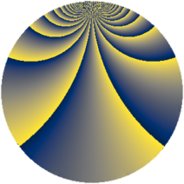# Properties

 Label 675.2.uLevel $675$ Weight $2$ Character orbit 675.u Rep. character $\chi_{675}(49,\cdot)$ Character field $\Q(\zeta_{18})$ Dimension $312$ Newform subspaces $5$ Sturm bound $180$ Trace bound $1$

# Related objects

## Defining parameters

 Level: $$N$$ $$=$$ $$675 = 3^{3} \cdot 5^{2}$$ Weight: $$k$$ $$=$$ $$2$$ Character orbit: $$[\chi]$$ $$=$$ 675.u (of order $$18$$ and degree $$6$$) Character conductor: $$\operatorname{cond}(\chi)$$ $$=$$ $$135$$ Character field: $$\Q(\zeta_{18})$$ Newform subspaces: $$5$$ Sturm bound: $$180$$ Trace bound: $$1$$ Distinguishing $$T_p$$: $$2$$

## Dimensions

The following table gives the dimensions of various subspaces of $$M_{2}(675, [\chi])$$.

Total New Old
Modular forms 576 336 240
Cusp forms 504 312 192
Eisenstein series 72 24 48

## Trace form

 $$312 q + 12 q^{4} - 24 q^{6} + 24 q^{9} + O(q^{10})$$ $$312 q + 12 q^{4} - 24 q^{6} + 24 q^{9} - 24 q^{11} + 24 q^{14} + 6 q^{19} - 48 q^{21} - 6 q^{24} - 96 q^{26} + 48 q^{29} + 6 q^{31} - 96 q^{36} + 30 q^{39} - 12 q^{41} - 102 q^{44} - 6 q^{46} + 48 q^{49} - 54 q^{51} - 192 q^{54} + 138 q^{56} + 42 q^{59} - 48 q^{61} + 120 q^{64} + 174 q^{66} - 60 q^{69} + 78 q^{71} - 138 q^{74} + 12 q^{76} - 60 q^{79} - 186 q^{84} - 42 q^{86} - 36 q^{89} - 6 q^{91} - 60 q^{94} - 168 q^{96} - 24 q^{99} + O(q^{100})$$

## Decomposition of $$S_{2}^{\mathrm{new}}(675, [\chi])$$ into newform subspaces

Label Dim $A$ Field CM Traces $q$-expansion
$a_{2}$ $a_{3}$ $a_{5}$ $a_{7}$
675.2.u.a $12$ $5.390$ $$\Q(\zeta_{36})$$ None $$0$$ $$0$$ $$0$$ $$0$$ $$q+(-\zeta_{36}^{5}+\zeta_{36}^{7}-\zeta_{36}^{9})q^{2}+(-2\zeta_{36}^{5}+\cdots)q^{3}+\cdots$$
675.2.u.b $24$ $5.390$ None $$0$$ $$0$$ $$0$$ $$0$$
675.2.u.c $60$ $5.390$ None $$0$$ $$0$$ $$0$$ $$0$$
675.2.u.d $84$ $5.390$ None $$0$$ $$0$$ $$0$$ $$0$$
675.2.u.e $132$ $5.390$ None $$0$$ $$0$$ $$0$$ $$0$$

## Decomposition of $$S_{2}^{\mathrm{old}}(675, [\chi])$$ into lower level spaces

$$S_{2}^{\mathrm{old}}(675, [\chi]) \cong$$ $$S_{2}^{\mathrm{new}}(135, [\chi])$$$$^{\oplus 2}$$